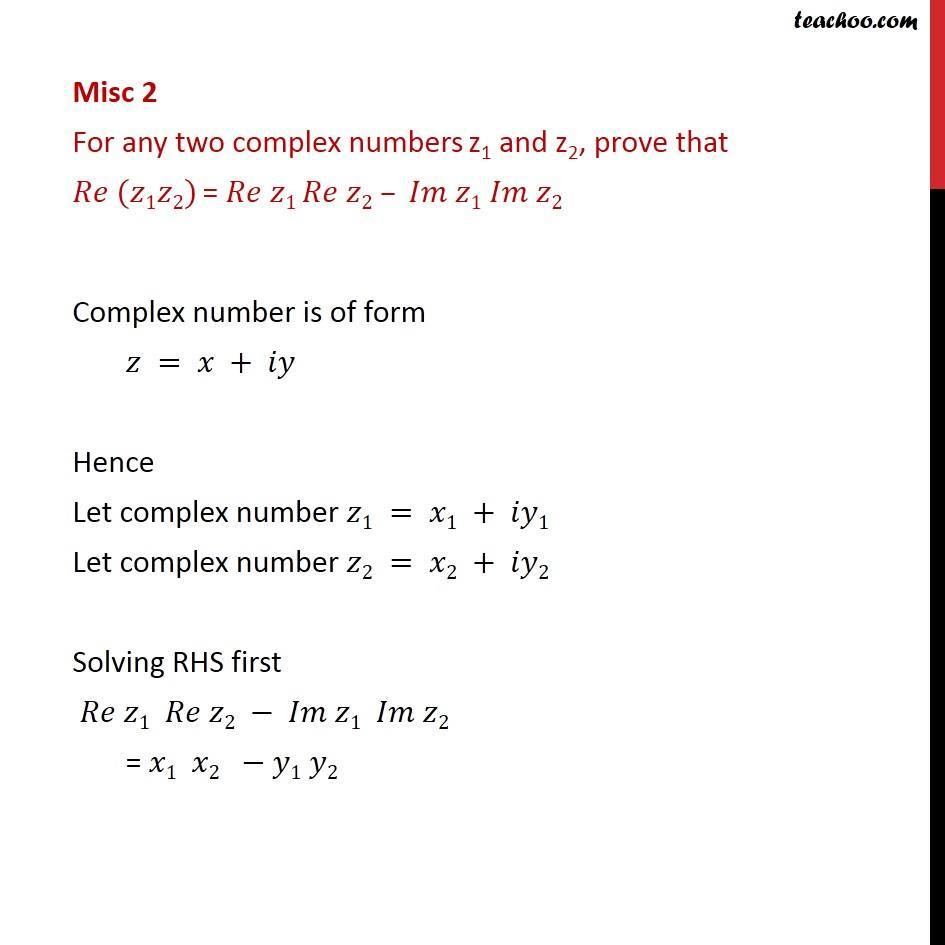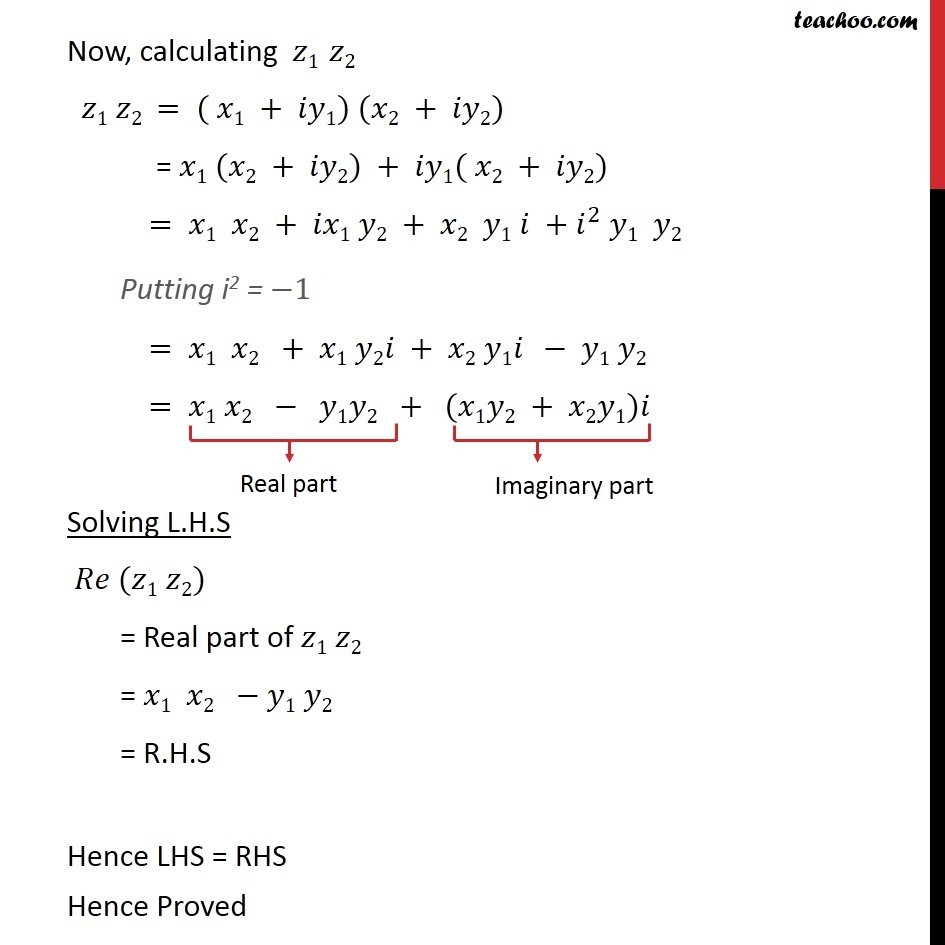Proof- Taking general complex numbers

Chapter 5 Class 11 Complex Numbers (Term 1)
Concept wise### Transcript

Misc 2 For any two complex numbers z1 and z2, prove that ( 1 2) = 1 2 1 2 Complex number is of form = + Hence Let complex number 1 = 1 + 1 Let complex number 2 = 2 + 2 Solving RHS first 1 2 1 2 = 1 2 1 2 Now, calculating 1 2 1 2 = ( 1 + 1) ( 2 + 2) = 1 ( 2 + 2) + 1( 2 + 2) = 1 2 + 1 2 + 2 1 + ^2 1 2 Putting i2 = 1 = 1 2 + 1 2 + 2 1 1 2 = 1 2 1 2 + ( 1 2 + 2 1) Solving L.H.S ( 1 2) = Real part of 1 2 = 1 2 1 2 = R.H.S Hence LHS = RHS Hence Proved